# Solving System Of Linear Equations Using Cramer’s Rule

Cramer’s rule is a method of solving the system of linear equations using determinants. It is named after Gabriel Cramer (1704–1752) who discovered this method. In this article we are going to discuss and work few examples for solving system of linear equations using Cramer’s rule.

Before you start reading about Cramer’s rule, learn to compute determinant of 2 x 2 and 3 x 3 matrix as a prerequisite to this article : Finding Determinants Using Cross Multiplication.

Coefficient Matrix and Augmented Matrix

Given a system of equation you can derive a coefficient matrix and an augmented matrix from it. Both are the same thing with little different.

Suppose you are given a system of linear equations with 2 unknowns.

\begin{aligned}
&a_{11}x + a_{12}y = b_1\\\\
&a_{21}x + a_{22}y = b_2
\end{aligned}

The augmented matrix and coefficient matrix would look like the following.

The system of linear equation is in the form of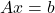which is written as

\begin{aligned}
&Ax = b\\\\
&\begin{bmatrix}a_{11} & a_{12}\\a_{21} & a_{22}\end{bmatrix} . \begin{bmatrix}x \\ y\end{bmatrix} = \begin{bmatrix}b_1 \\ b_2\end{bmatrix}
\end{aligned}

The matrix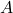is coefficient matrix,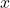is a column vector of unknowns, and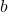represents the constant vector.

Determinant Of 2 x 2 Matrix

The determinant of a 2 x 2 matrix can be calculated using cross multiplication.

\begin{aligned}
&A = \begin{bmatrix}a_{11} & a_{12}\\a_{21} & a_{22}\end{bmatrix}\\\\
&|A| = \begin{vmatrix}a_{11} & a_{12}\\a_{21} & a_{22}\end{vmatrix} = a_{11}a_{22} - a{12}.a_{21}
\end{aligned}

Example #1

Find the determinant of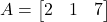.

Solution:

|A|= \begin{vmatrix}2 & 1\\3 & 7\end{vmatrix} = 14 - 3 = 11

### Solving System of Equations with 2 Unknowns With Cramer’s Rule

Given a system of equations with 2 unknowns you can solve it using Cramer’s rule by following steps.

1. Find the augmented matrix and the coefficient matrix for the system of equations.
2. Find the determinant of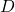of matrix.
3. Replace thecoefficients of coefficient matrixwith constant vectorto get x-matrix and find its determinant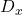4. Replace the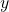coefficients of coefficient matrixwith constant vectorto get y-matrix and find its determinant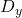5. Find the solution to system of linear equation according to following equations.
\begin{aligned}
&x = D_x/D\\\\
&y = D_y/D
\end{aligned}

See the following figure to understand how the matrices look like.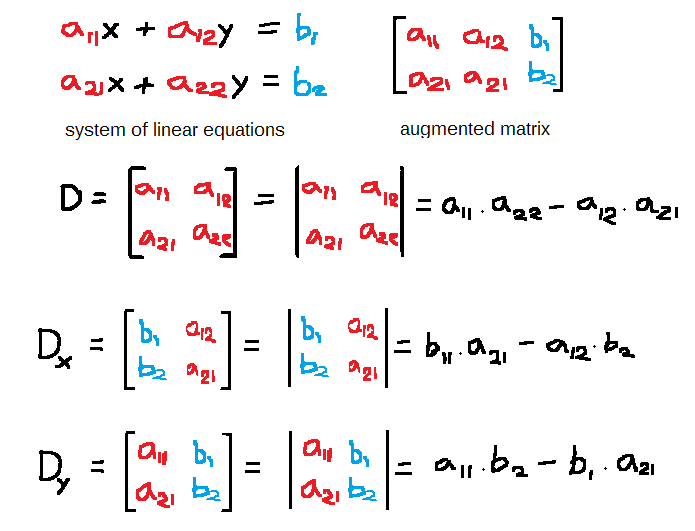Figure1- Determinant of x-matrix and y-matrix must be obtained along with determinant of 2 x 2 matrix.

Given a system of equations such as shown above, first derive the augmented matrix and coefficient matrix. The coefficient matrix has no constant vector. Use the coefficient matrix to get the determinant of matrixwhich is.

X Matrix And Y Matrix

You can get x matrix by replacing the first column which is x column by the constant vector. See the image below.

Similarly, compute the y matrix from coefficient matrix of. Compute the determinant of x matrix which isand compute the determinant of y matrix which is.

To find the solution to each unknowns use the following formula, mentioned earlier.

Solution for x

\begin{aligned}
&x = D_x/D
\end{aligned}

Solution for y,

y = D_y/D

In the next section, we will solve a system of linear equation with two unknown.

Example #1

Find the solution to following system of linear equations with two unknowns using Cramer’s rule.

\begin{aligned}
&x + 3y = 5\\\\
&5x - 2y = 8
\end{aligned}

Solution:

Given the system of linear equations we can obtain augmented matrix and coefficient matrix.

A = \begin{bmatrix}1 & 3 & 5\\5 & -2 & 8\end{bmatrix}

The coefficient matrix must be a square matrix, so lets write the augmented matrix in the formin that order.

\begin{aligned}
&Ax = b\\\\
&\begin{bmatrix}1& 3\\5 & -2\end{bmatrix} . \begin{bmatrix}x & y\end{bmatrix} = \begin{bmatrix}5 & 8\end{bmatrix}
\end{aligned}

Compute the determinant of the matrix.

D = \begin{vmatrix}1& 3\\5 & -2\end{vmatrix} = -2 - 15 = -17

Compute the determinant of thematrix.

D_x = \begin{vmatrix}5 & 3\\8 & -2\end{vmatrix} = -10 - 24 = -34

Compute the determinant of thematrix.

D_y = \begin{vmatrix}1& 5\\5 & 8\end{vmatrix} = 8 - 25 = -17

Therefore,

\begin{aligned}
&x = -34/(-17) = 2\\\\
&y = -17/(-17)= 1
\end{aligned}

We can verify the solution by substitution.

\begin{aligned}
&(2) + 3(1) = 5\\\\
&5(2) - 2(1) = 8
\end{aligned}

The solutions to the system of linear equations are correct.

### Solving System Of Equations With 3 Unknowns Using Cramer’s Rule

We can solve the system of equations with 3 unknowns which gives a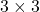square matrix using the Cramer’s rule.

Though we need to compute the determinant of additional sub-matrices because there is one more unknown other than x and y. Let us call it z.

The steps remain the same as we solved for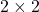matrix. Here is the list of steps.

Letbe amatrix.

Step 1: Compute determinantof.

Step 2: You must extract sub-matrices or minors from the matrixand take determinant